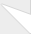Warning: INSERT command denied to user 'dbo292345962'@'74.208.59.105' for table 'watchdog' query: INSERT INTO watchdog (uid, type, message, variables, severity, link, location, referer, hostname, timestamp) VALUES (0, 'php', '%message in %file on line %line.', 'a:4:{s:6:\"%error\";s:12:\"user warning\";s:8:\"%message\";s:400:\"INSERT command denied to user &#039;dbo292345962&#039;@&#039;74.208.59.105&#039; for table &#039;captcha_sessions&#039;\nquery: INSERT into captcha_sessions (uid, sid, ip_address, timestamp, form_id, solution, status, attempts) VALUES (0, &#039;faeba75d56586568017bbeb90dea607e&#039;, &#039;3.234.241.200&#039;, 1594305808, &#039;comment_form&#039;, &#039;a8464f107572f2675a8804f0944f681a&#039;, 0, 0)\";s:5:\"%file\";s:62:\"/homepages/25/d199835659/htdocs/ID/modules/captcha/captcha.inc\";s:5:\"%line\";i:99;}& in /homepages/25/d199835659/htdocs/ID/includes/database.mysql.inc on line 135
Simple C++ Pointers and References | Inferno Development

# Simple C++ Pointers and References

Pointers are used to point to a variable rather than copying the variable all over your functions and classes. Usually when you pass a variable to a function, it copies the variable wasting memory.

### C++ Pointers

By using pointers, you can just send an address of the variable so that the function changes the variable directly rather than changing the copy of the variable.

The easiest way to think of pointers, is to think of an index card that you show to your friend that tells him where the full article is (or variable in our case). When you see the * (asterisk) symbol, it means that it is a pointer, literally translating as "address "

If you declare a variable and then you want to use a pointer to point to that variable, when you copy your variable to the pointer you use & (ampersand character) to indicate that you don't want to copy the variable, but the address to the variable to the pointer.

The easiest way to think of a reference is to think of an index card, but the title is the reference not the index card. It is the same thing as referencing a document in an essay. A reference & symbol can also be translated as "The address of ".

Let's see an example:

int i, final;
i = 500;
int Calculate(int v){
v += 100;
return v;
}
final = Calculate(i);

Now integer final is equal to 600. However, we had to copy i to v, and then to final.
How can we improve this function using pointers and references?

int i;
int* final;
i = 500;
void Calculate(int *v){
*v += 100;
}
Calculate(&i);

Same process, except we used * to indicate a pointer, and & to reference or "give the address of" variable i. In this example, we used an int datatype and used pointers with it.

However, using pointers with int is actually slower than directly copying it, so I don't recommend using pointers with int. This method of using pointers works well with other data types or classes and structs; however, using int in this example will help explain the concept better.

We also did *v += 100, instead of v += 100, because v += 100, will increase the pointer (which means increasing the memory address); using *v means we want the actual value that it is pointing to.

Let's try that with char.

#include <stdio.h>
char Car;
sprintf(Car, "Toyota Camry");
int GetCar(char *vehicle){
strncat(vehicle, " Year: 2007 hybrid", 18); // strncat for more security
return 1;
}
GetCar(Car);
printf("%s", Car);

This will print: Toyota Camry Year: 2007 hybrid

All we did was change the referenced variable Car, without actually returning it from the function. We changed the referenced char Car, from inside the function, although we would normally use & when passing Car to GetCar, but it is not needed in this situation.

Using the pointer vehicle, it became a small variable that holds the address of Car, so in essence, vehicle and Car are one in the same in this example.

References in parameters can be used similar to pointers, the following function takes a reference of its parameter and multiply it by five.

void MultiplyByFive(int& number){
number *= 5;
}

iGlobal += Num;
}

These two functions can be used like this:

int x = 10;
MultiplyByFive(x);
cout << "X is now: " << x << endl; // X is now: 50
cout << "iGlobal is now: " << iGlobal << endl; // iGlobal is now added
// with 50

int& number can be used to get a pointer to x and multiply it by 5 without returning any value.

const int& Num, can be used to pass a variable WITHOUT copying the variable like normal parameters; you cannot edit a const int&.

References can also be used mostly similar to the const int&, with many data types. It can increase the efficiency of your programs.

### Summary

Here is a small summary to remember pointers and references easily:

int a(50);
int *pointer = &a; /* Declared pointer "pointer", set it to look at the "address of" (&) integer a. */
*pointer = 20; /* I just SET a = 20! */
MultiplyByFive(a);

### Thanks for giving notesThanks for giving notes